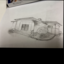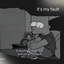Mathematics 25 OnlineOpenStudy (anonymous):

Find an equation of the line satisfying the conditions given. Express your answer in standard form. Parallel to 3x - y = -5 and passing through ( -1, 0 )OpenStudy (bahrom7893):

If it is parallel to the line 3x - y = -5, then it must have the same slope as that line: Let me rewrite your equation, so that it is easier for me to see the slope: 3x - y = -5, move 3x to the right hand side: - y = - 5 - 3x, multiply thru by -1: y = 5 + 3x or y = 3x +5. This is in the form of y = Mx + b, where M = slope = 3OpenStudy (bahrom7893):

Okay so now the formula of the line is: \[y - y _{1} = M ( x - x _{1})\] In your case: \[x_{1} = -1\] and \[y_{1} = 0\] ( -1 ; 0 )OpenStudy (bahrom7893):

so you know that M = 3; x1 = -1 and y1 = 0. Plug these into the equation of a line above: y - ( 0 ) = 3 * ( x - (-1) ) Simplify: y = 3x + 3 <=Your answerOpenStudy (bahrom7893):

any questions?

Latest Questionsineedagfsobad122: hey what ever ur name is on the vc girl i think whats ur qc if its u text back
1 hour ago 1 Reply 0 MedalsTbone: Edits ^-^
40 seconds ago 5 Replies 3 Medalsrose12345: look
5 hours ago 11 Replies 3 Medalsjessii: What are the pros and cons of being a flight attendant?
3 hours ago 4 Replies 0 MedalsSamRoman: What do y'all think of my Cover photo for my next YouTube video?
6 hours ago 11 Replies 1 Medallovelygirl13: i made a collage of the color green
3 hours ago 4 Replies 1 MedalAlexisWolfe: 1. At the beginning of Chapter 3, Holden says, u201cIu2019m the most terrific liar you ever saw in your life.
9 hours ago 0 Replies 0 Medalschrishale13840: would this be a good tattoo
9 hours ago 18 Replies 5 MedalsZayne2003: Why was everyone there at the Tabard inn (beginning of Canterbury Tales)?
9 hours ago 8 Replies 1 Medal